# Algebra 1 : How to find the whole from the part with percentage

## Example Questions

1 3 Next →

### Example Question #21 : How To Find The Whole From The Part With Percentage

What number ofis nine?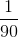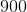Explanation:

Let the number be.

Some number of ten percent means some number multiplied by ten percent.

A percentage is a number out of 100 parts.

Set up the equation.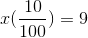Reduce the fraction and simplify the left side.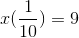Multiply both sides by ten to eliminate the fraction.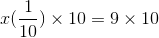Simplify both sides.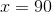The answer is.

### Example Question #22 : How To Find The Whole From The Part With Percentage

What number of twenty percent is 40?Explanation:

Write an equation to express the percentage and the part to find the whole.

A percentage is a number out of 100 parts.  Theis the whole that we will need to find.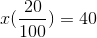Reduce the fraction.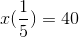Multiply by five on both sides.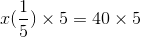Simplify the left and right sides.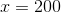The answer is.

### Example Question #23 : How To Find The Whole From The Part With Percentage

What number of twelve percent is four?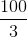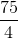Explanation:

Set up an equation to represent this scenario.

Let the number be.

A percentage is some number out of 100 parts.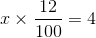Multiply both sides by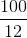.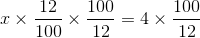Simplify both sides.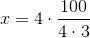The answer is:### Example Question #24 : How To Find The Whole From The Part With Percentage

What number of five percent is ten?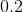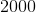Explanation:

Set up the equation so that some number multiplied with five percent is equal to ten.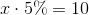The percentage can be rewritten as a fraction.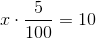Simplify the fraction.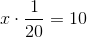Multiply by the denominator on both sides to eliminate the fraction.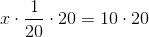Simplify both sides.

The answer is:### Example Question #25 : How To Find The Whole From The Part With Percentage

What number of forty percent is six?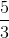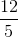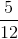Explanation:

Write an equation such that some number times 40 parts of 100 is equal to six.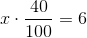Reduce the fraction.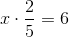To isolate the x-variable, multiply both sides by the reciprocal of the fraction.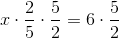Simplify both sides.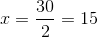The answer is:### Example Question #26 : How To Find The Whole From The Part With Percentage

In a classroom survey, 18 kids reported having brown hair. The teacher calculated that the 18 with brown hair make up 60% of her class. How many total students are in the class?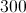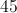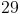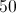Explanation:

Set up an equation representing the information you are given to start (18 out of an unknown total equals 60% or 60 out of 100).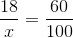Manipulate the equation to get X by itself.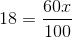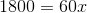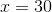There are 30 total students in the class.

### Example Question #27 : How To Find The Whole From The Part With Percentage

Nine is fifty percent of what number?Explanation:

Set up an equation such that a number times 50 out of 100 parts is nine.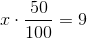Solve this equation.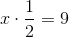Multply by two on both sides to eliminate the fraction.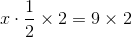Simplify both sides.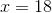1 3 Next →